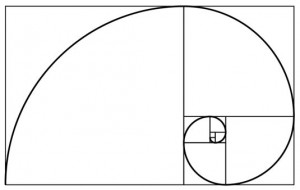# 1

• It is the multiplicative identity for integers, real numbers, and complex numbers.
• It is neither prime nor composite
• 1 raised to any number is 1.
• Is considered a highest honor. 1 is #1!

# 1.61803398874989484820…

• AKA phi
• Φ
• It is the “Golden Ratio”
• It is found by dividing a line into two parts, so that the longer part divided by the smaller part is also equal to the whole length divided by the longer part:
a/b=(a+b)/a
• The actual value goes infinitely with no pattern.
• It is an irrational number, which means it cannot be written as the ratio of two integers# 3.14159265358979323846…

• AKA pi
• Π
• It is the ratio of a circle’s circumference to its diameter.
• It is an irrational number.
• It has an infinite number of digits in its decimal representation and does not settle into any repeating pattern.# 6

• It is the first perfect number. The sum of all of its divisors:
1+2+3=6
• It is the only number that is both the sum and the product of the same three consecutive positive integers :
1+2+3=1*2*3
• It is the atomic number of carbon. Carbon is the basis of almost all known life on Earth.
• It has significance in both Christian and Jewish religion.